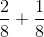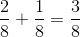SSAT Middle Level Math : How to add fractions

Example Questions

Example Question #111 : How To Add Fractions

Lily pulledof the weeds and Rose pulled. What fraction of the weeds have they pulled?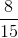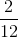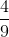Explanation: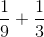In order to solve this problem, we first need to make common denominators.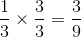Now that we have common denominators, we can add the fractions. Remember, when we add fractions, the denominator stays the same, we only add the numerator.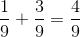Example Question #1 : Adding Fractions

Select the fraction model that shows the sum of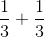Explanation: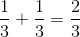The fraction model is broken up into three pieces and two of the pieces are shaded in. The numerator of the fraction tells us how many pieces should be shaded in, and the denominator tells us how many pieces the whole should be split up into.

Example Question #2 : Understand Addition And Subtraction Of Fractions: Ccss.Math.Content.4.Nf.B.3a

Select the fraction model that shows the sum of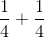Explanation: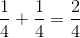The fraction model is broken up into four pieces and two of the pieces are shaded in. The numerator of the fraction tells us how many pieces should be shaded in, and the denominator tells us how many pieces the whole should be split up into.

Example Question #113 : How To Add Fractions

Select the fraction model that shows the sum of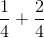Explanation: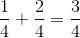The fraction model is broken up into four pieces and three of the pieces are shaded in. The numerator of the fraction tells us how many pieces should be shaded in, and the denominator tells us how many pieces the whole should be split up into.

Example Question #311 : Build Fractions From Unit Fractions

Select the fraction model that shows the sum of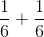Explanation: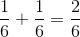The fraction model is broken up into six pieces and two of the pieces are shaded in. The numerator of the fraction tells us how many pieces should be shaded in, and the denominator tells us how many pieces the whole should be split up into.

Example Question #312 : Build Fractions From Unit Fractions

Select the fraction model that shows the sum of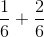Explanation: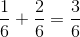The fraction model is broken up into six pieces and three of the pieces are shaded in. The numerator of the fraction tells us how many pieces should be shaded in, and the denominator tells us how many pieces the whole should be split up into.

Example Question #313 : Build Fractions From Unit Fractions

Select the fraction model that shows the sum of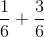Explanation: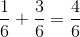The fraction model is broken up into six pieces and four of the pieces are shaded in. The numerator of the fraction tells us how many pieces should be shaded in, and the denominator tells us how many pieces the whole should be split up into.

Example Question #314 : Build Fractions From Unit Fractions

Select the fraction model that shows the sum of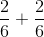Explanation: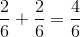The fraction model is broken up into six pieces and four of the pieces are shaded in. The numerator of the fraction tells us how many pieces should be shaded in, and the denominator tells us how many pieces the whole should be split up into.

Example Question #315 : Build Fractions From Unit Fractions

Select the fraction model that shows the sum of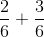Explanation: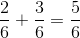The fraction model is broken up into six pieces and five of the pieces are shaded in. The numerator of the fraction tells us how many pieces should be shaded in, and the denominator tells us how many pieces the whole should be split up into.

Example Question #316 : Build Fractions From Unit Fractions

Select the fraction model that shows the sum of